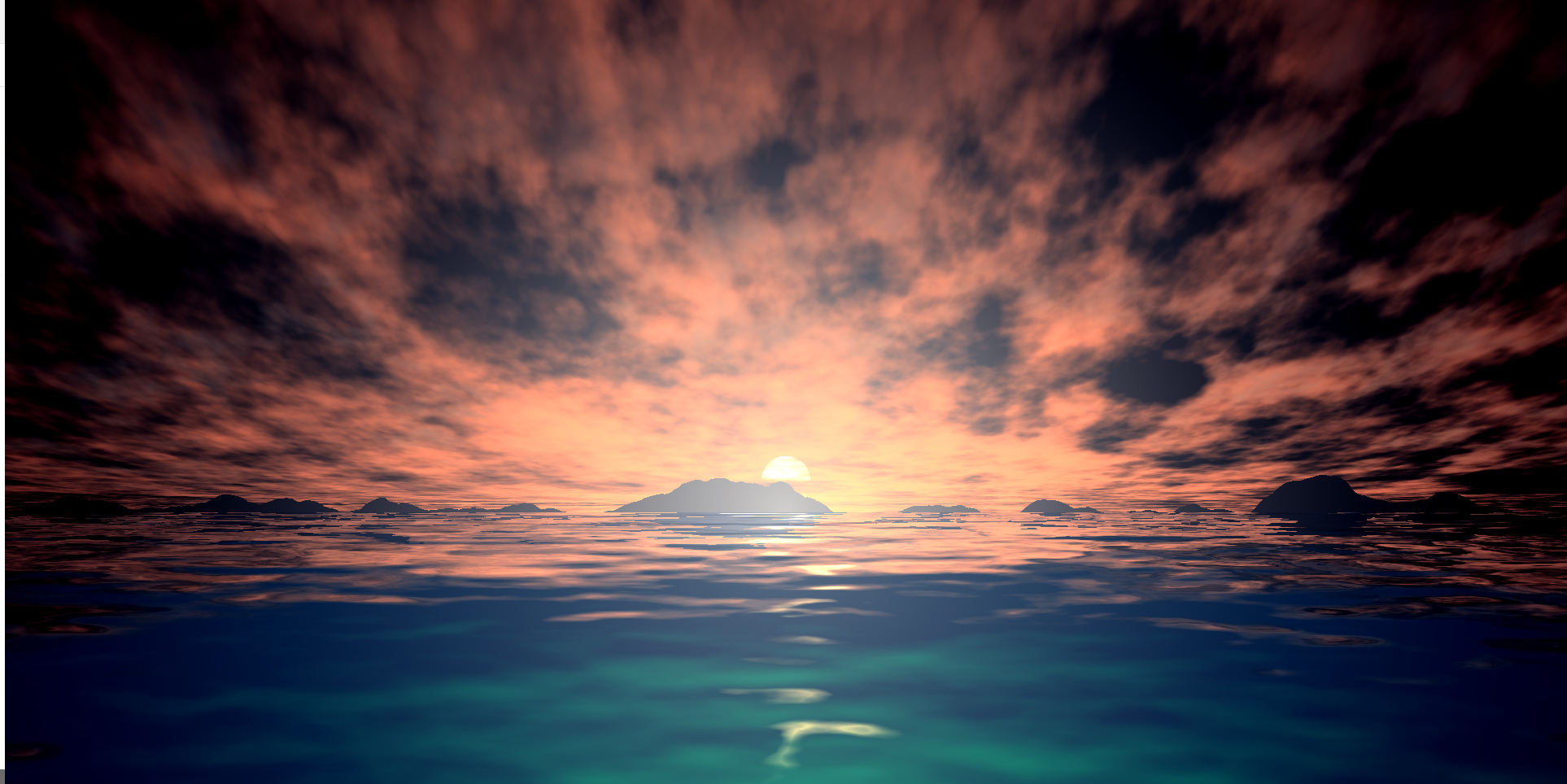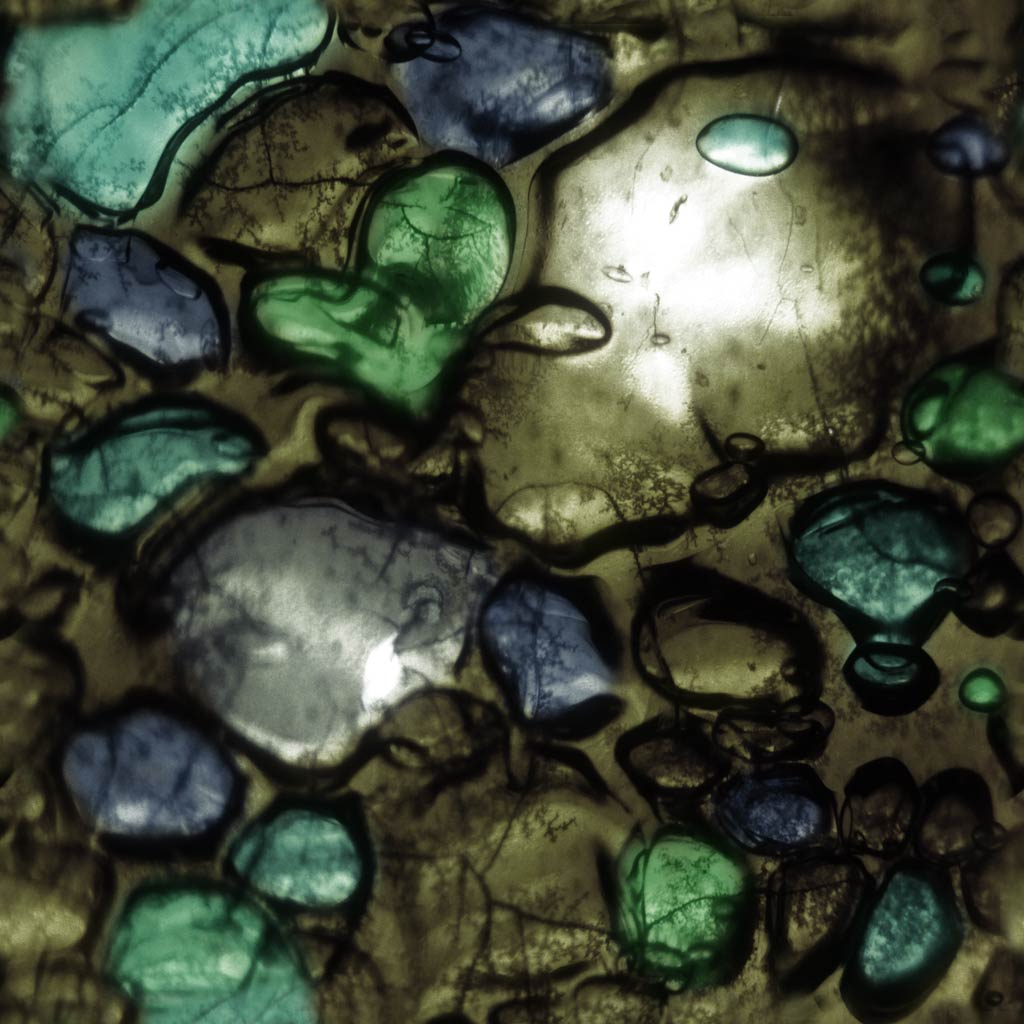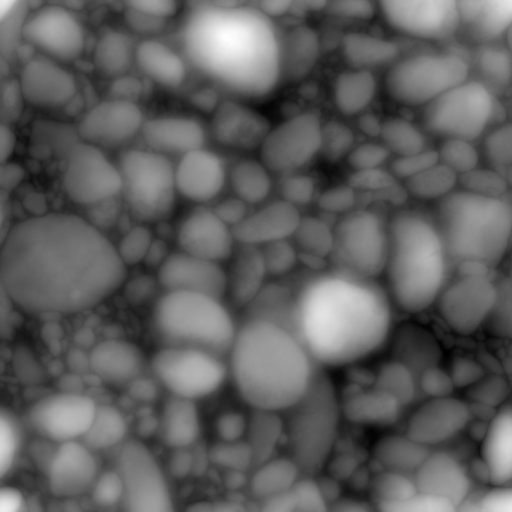• 下面是shader代码，在webgl可以运行，在three也可以 <script id="vertexshader" type="x-shader/x-vertex"> attribute vec3 A_position3; attribute vec2 A_uv; uniform mat4 mvp; varying vec2 out_uv; ...
shdertoy上的动态黄昏图
看效果外加两张shader里用到的图片<script id="vertexshader" type="x-shader/x-vertex">
attribute vec3 A_position3;
attribute vec2 A_uv;
uniform mat4 mvp;

varying vec2 out_uv;

void main(void){

gl_Position = mvp * vec4(A_position3,1.0);

out_uv = A_uv;
}
</script>
precision highp float;
precision highp int;
varying vec2 out_uv;
uniform vec2 iResolution;
uniform float iTime;
uniform sampler2D iChannel0;
uniform sampler2D iChannel1;

const vec3 sunColor = vec3(1.5,.9,.7);
const vec3 lightColor = vec3(1.,.8,.7);
const vec3 darkColor = vec3(.2,.2,.3);
const vec3 baseSkyColor = vec3(.6,.7,.8);
const vec3 seaColor = vec3(.1,.3,.5);
const vec3 seaLight = vec3(.1,.45,.55);
const vec2 iMouse = vec2(60.0);
//---------------------------------------

vec3 gamma( vec3 col, float g){
return pow(col,vec3(g));
}

// clouds layered noise
float noiseLayer(vec2 uv){
float t = (iTime+iMouse.x)/5.;
uv.y -= t/60.; // clouds pass by
float e = 0.;
for(float j=1.; j<9.; j++){
// shift each layer in different directions
float timeOffset = t*mod(j,2.989)*.02 - t*.015;
e += 1.-texture2D(iChannel0, uv * (j*1.789) + j*159.45 + timeOffset).r / j ;
}
e /= 3.5;
return e;
}

// waves layered noise
float waterHeight(vec2 uv){
float t = (iTime+iMouse.x);
float e = 0.;
for(float j=1.; j<6.; j++){
// shift each layer in different directions
float timeOffset = t*mod(j,.789)*.1 - t*.05;
e += texture2D(iChannel1, uv * (j*1.789) + j*159.45 + timeOffset).r / j ;
}
e /= 6.;
return e;
}

vec3 waterNormals(vec2 uv){
uv.x *= .25;
float eps = 0.008;
vec3 n=vec3( waterHeight(uv) - waterHeight(uv+vec2(eps,0.)),
1.,
waterHeight(uv) - waterHeight(uv+vec2(0.,eps)));
return normalize(n);
}

vec3 drawSky( vec2 uv, vec2 uvInit){

float clouds = noiseLayer(uv);

// clouds normals
float eps = 0.1;
vec3 n = vec3(	clouds - noiseLayer(uv+vec2(eps,0.)),
-.3,
clouds - noiseLayer(uv+vec2(0.,eps)));
n = normalize(n);

// fake lighting
float l = dot(n, normalize(vec3(uv.x,-.2,uv.y+.5)));
l = clamp(l,0.,1.);

// clouds color	(color gradient from light)
vec3 cloudColor = mix(baseSkyColor, darkColor, length(uvInit)*1.7);
cloudColor = mix( cloudColor,sunColor, l );

// sky color (color gradient on Y)
vec3 skyColor = mix(lightColor , baseSkyColor, clamp(uvInit.y*2.,0.,1.) );
skyColor = mix ( skyColor, darkColor, clamp(uvInit.y*3.-.8,0.,1.) );
skyColor = mix ( skyColor, sunColor, clamp(-uvInit.y*10.+1.1,0.,1.) );

// draw sun
if(length(uvInit-vec2(0.,.04) )<.03){
skyColor += vec3(2.,1.,.8);
}

// mix clouds and sky
float cloudMix = clamp(0.,1.,clouds*4.-8.);
vec3 color = mix( cloudColor, skyColor, clamp(cloudMix,0.,1.) );

// draw islands on horizon
uvInit.y = abs(uvInit.y);
float islandHeight = texture2D(iChannel1, uvInit.xx/2.+.67).r/15. - uvInit.y + .978;
islandHeight += texture2D(iChannel1, uvInit.xx*2.).r/60.;
islandHeight = clamp(floor(islandHeight),0.,1.);
vec3 landColor = mix(baseSkyColor, darkColor, length(uvInit)*1.5);
color = mix(color, landColor, islandHeight);

return color;
}
void main(void){
// center uv around horizon and manage ratio
vec2 uvInit = out_uv;
uvInit.x -= .5;
uvInit.x *= iResolution.x/iResolution.y;
uvInit.y -= 0.35;

// perspective deform
vec2 uv = uvInit;
uv.y -=.01;
uv.y = abs(uv.y);
uv.y = log(uv.y)/2.;
uv.x *= 1.-uv.y;
uv *= .2;

vec3 col = vec3(1.,1.,1.);

// draw water
if(uvInit.y < 0.){

vec3 n = waterNormals(uv);

// draw reflection of sky into water
vec3 waterReflections = drawSky(uv+n.xz, uvInit+n.xz);

// mask for fore-ground green light effect in water
float transparency = dot(n, vec3(0.,.2,1.5));
transparency -= length ( (uvInit - vec2(0.,-.35)) * vec2(.2,1.) );
transparency = (transparency*12.+1.5);

waterReflections = mix( waterReflections, seaColor, clamp(transparency,0.,1.) );
waterReflections = mix( waterReflections, seaLight, max(0.,transparency-1.5) );

col = waterReflections;

// darken sea near horizon
col = mix(col, col*vec3(.6,.8,1.), -uv.y);

//sun specular
col += max(0.,.02-abs(uv.x+n.x))* 8000. * vec3(1.,.7,.3) * -uv.y * max(0.,-n.z);

}else{

// sky
col = drawSky(uv, uvInit);
}

// sun flare & vignette
col += vec3(1.,.8,.6) * (0.55-length(uvInit)) ;

col *= .75;
col = gamma(col,1.3);
gl_FragColor = vec4(col,1.0);

}
</script>

展开全文WebGLShader 可以是一个顶点着色器（vertex shader）或片元着色器（fragment shader）。每个 WebGLProgram 都需要这两种类型的着色器。
function createShader (gl, sourceCode, type) {

throw "Could not compile WebGL program. \n\n" + info;
}
}

创建一个顶点着色器
var fragmentShaderSource =
"void main() {\n"+
"  gl_FragColor = vec4(1.0, 1.0, 1.0, 1.0);\n"+
"}\n";



展开全文可视化
• U3D frac 即 WebGL 内置函数 fract 返回x的小数部分 float frac(float x) { return x-floor(x); } //线性插值 float lerp(float from,float to, float t ) { t = max(0.0, min(1.0, t)); return from + ( to...
之前发过一些相关帖子 就懒得整理了 为什么呢?(因为懒 哈哈哈) 今后如果有需要补充的就放在这里了!
U3D frac 即 WebGL 内置函数 fract 返回x的小数部分
float frac(float x)
{
return x-floor(x);
}

//线性插值
float lerp(float from,float to, float t )
{
t = max(0.0, min(1.0, t));
return from + ( to - from ) * t;
}
vec3 lerp(vec3 from,vec3 to, float t )
{
t = max(0.0, min(1.0, t));
vec3 tmp;
tmp.x = from.x + ( to.x - from.x ) * t;
tmp.y = from.y + ( to.y - from.y ) * t;
tmp.z = from.z + ( to.z - from.z ) * t;
return tmp;
}
vec4 lerp(vec4 from,vec4 to, float t )
{
t = max(0.0, min(1.0, t));
vec4 tmp;
tmp.x = from.x + ( to.x - from.x ) * t;
tmp.y = from.y + ( to.y - from.y ) * t;
tmp.z = from.z + ( to.z - from.z ) * t;
tmp.w = from.w + ( to.w - from.w ) * t;
return tmp;
}
//返回0-1之间的数
float saturate(float x)
{
return max(0.0, min(1.0, x));
}
vec3 saturate(vec3 v)
{
v.x = max(0.0, min(1.0, v.x));
v.y = max(0.0, min(1.0, v.y));
v.z = max(0.0, min(1.0, v.z));
return v;
}
mul=matrixCmpMult  这两个是等效的
展开全文javascript typescript
• WebGL & GLSL & CSS ShadersWebGLWebGL是一种3D绘图标准，这种绘图技术标准允许把JavaScript和OpenGL ES 2.0结合在一起，通过增加OpenGL ES 2.0的一个JavaScript绑定，WebGL可以为HTML5 Canvas提供硬件3D加速渲染，...
WebGL & GLSL & CSS Shaders
WebGL
WebGL是一种3D绘图标准，这种绘图技术标准允许把JavaScript和OpenGL ES 2.0结合在一起，通过增加OpenGL ES 2.0的一个JavaScript绑定，WebGL可以为HTML5 Canvas提供硬件3D加速渲染，这样Web开发人员就可以借助系统显卡来在浏览器里更流畅地展示3D场景和模型了，还能创建复杂的导航和数据视觉化。显然，WebGL技术标准免去了开发网页专用渲染插件的麻烦，可被用于创建具有复杂3D结构的网站页面，甚至可以用来设计3D网页游戏等等。
由于webgl是基于OpenGL和JavaScript技术结合，而OpenGL与微软DirectX存在着竞争关系，而且微软自身也开发了Silverlight插件，与webgl其实是类似的，所以微软对webgl技术并不支持。这很好理解，好比五阿哥和尔康在争夺心爱的容嬷嬷之前是不可能相互拥抱的。
微软的不支持并不影响webgl的发展，随着firefox、谷歌和苹果等加入，webgl也开始出现了各种应用。
GLSL

简而言之，css shaders 让 css技术 具有 3D图形 特性

在CSS中使用着色器可以制作出很多吸引眼球的动态效果。
Adobe展示了一些Demo视频，在第一个视频中Twitter的页面被渲染成了弯曲的表面；第二个视频更加有趣，将Google地图和街景渲染折叠了起来，像真的纸质地图那样。因为着色器可以作用于所有HTML内容，所以CSS Shaders渲染的元素包括了普通的表格、SVG甚至是HTML5 Video等等。
展开全文css 前端egret LayaboxLayaBox
• 交互过程产生的阳光和天空。 运行它。 这从我的Ludum Dare 33条目扩展了代码。 用法 使用演示页面右上方的控件来修改着色器变量并创建自己的天空。 屏幕截图 学分 该项目用编写，使用： ... Yaroslav Sivakov的 ...
• glo着色器 WebGL的通用着色器接口。 与模块一起理想地有用，但不专门与它们绑定。 用法 执照 麻省理工学院，有关详细信息，请参阅 。JavaScript
• 合理的方案是：单独在可视化编辑器里可以单写，在场景文件中也以单独文件形式存在，导出场景文件在Asset Pipeline里编译时应该以字符串的形式编译进Asset里，和普通3d引擎是一样的，不要直接写进html里，更不要在...js
• WebGL 着色器的包装器。 例子 现在在您的浏览器中尝试一下： : var shell = require ( 'gl-now' ) ( ) var createShader = require ( 'gl-shader' ) var shader , buffer shell . on ( 'gl-init' , function ( ) ...JavaScript
• WebGL Shader骑师（ts版） 可视化 这些是应用程序的骨干。 每个可视化都有一个类，每个可视化处理其所有制服。 BaseVisualization任何可视化的基础。 处理更新源。 必须对其进行扩展，并且必须覆盖meshObservable()...JavaScript
• WebGLProgram 是 WebGL API 的一部分，它由两个WebGLShaders （webgl 着色器）组成，分别为顶点着色器和片元着色器（两种着色器都是采用 GLSL 语言编写的）。创建一个 WebGLProgram 需要调用 GL 上下文的...
• var canvasElement=document.getElementById('webgl');  var gl=canvasElement.getContext('webgl');  //顶点着色器源码  var vertexShaderSource = '' +  'attribute vec4 a_Position;'+//顶点位置坐标  ...
• 返回0.0-1.0之间的数 float saturate(float x) { return max(0.0, min(1.0, x)); }javascript typescriptglsl JavaScript
• 正如《WebGL 编程指南》中所说的： 传统的三维图形程序通常使用 C 或 C++ 等语言开发，并为特定的平台被编译成二进制的可执行文件。这意味着程序不能跨平台运行。相比之下，WebGL 程序由 HTML 和 JavaScript 组成，...
• webgl-着色器-dj 使用 glsl 着色器进行视觉 djing 的 Web 应用程序。JavaScriptopenglJavaScript
• WebGL着色器查看器 这是用于预览着色器文件的Visual Studio Code扩展。 特征 在VSC Web视图中显示指定着色器的预定义或... 在vscode中打开命令面板，然后运行“打开WebGL Shader Viewer” 选择顶点和片段着色器 执照TypeScript

千次阅读 2011-11-10 09:20:18matrix buffer 存储threejs3Dthree.js...